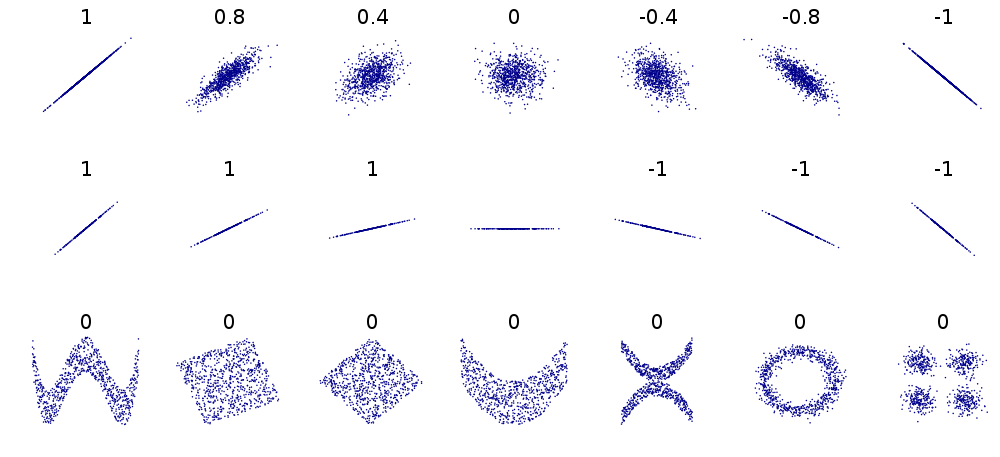Welcome to Project Lovelace! We're still in early development so there are still tons of bugs to find and improvements to make. If you have any suggestions, complaints, or comments please let us know on Discourse, Discord, or GitHub!

# Correlation does not imply causation

## You will learn about: statistics and lists, and summing lots of numbers.

Two variables are correlated if there's some statistical relationship between the two. However, just because two variables are correlated does not mean that one is caused by the other. This misconception is commonly referred to as “correlation does not imply causation”.Correlation coefficients $r$ for 24 different example data sets $(x, y)$. Top row: values of $r$ close to -1 and 1 suggest very linear relationships with little spread while $r$ close to 0 suggest no relationship and a lot of spread. Middle row: $r>0$ values suggest positive correlations while $r<0$ suggest negative correlations. Bottom row: many nonlinear relationships result in $r=0$ suggesting that the Pearson correlation coefficient is only good for measuring linear relationships. (Image credit: DenisBoigelot, Wikimedia Commons)

One way of computing a correlation coefficient between two variables $X$ and $Y$ with $n$ measurements $x_1, x_2, \dots, x_n$ and $y_1, y_2, \dots, y_n$ is the Pearson correlation coefficient $$r = \frac{\operatorname{cov}(X,Y)}{\sigma_X\sigma_Y}$$ where $$\operatorname{cov}(X,Y) = \frac{1}{n} \sum_{i=1}^n (x_i - \overline{x})(y_i - \overline{y}) = \frac{1}{n} \left[ (x_1-\overline{x})(y_1-\overline{y}) + \cdots + (x_n-\overline{x})(y_n-\overline{y}) \right]$$ is the covariance between $X$ and $Y$, \begin{align} \sigma_X & = \sqrt{\frac{1}{n} \sum_{i=1}^n (x_i - \overline{x})^2} = \sqrt{\frac{1}{n} \left[ (x_1 - \overline{x})^2 + \cdots + (x_n-\overline{x})^2 \right]} \quad \text{and} \\ \sigma_Y & = \sqrt{\frac{1}{n} \sum_{i=1}^n (y_i - \overline{y})^2} = \sqrt{\frac{1}{n} \left[ (y_1 - \overline{y})^2 + \cdots + (y_n-\overline{y})^2 \right]} \end{align} are the standard deviations of $X$ and $Y$, and $$\overline{x} = \frac{1}{n} \sum_{i=1}^n x_i = \frac{x_1 + x_2 + \cdots + x_n}{n} \quad \text{and} \quad \overline{y} = \frac{1}{n} \sum_{i=1}^n y_i = \frac{y_1 + y_2 + \cdots + y_n}{n}$$ are the averages (or means) of the $X$ and $Y$ measurements. The Pearson correlation coefficient $r$ is always between -1 and 1.

Taking in two lists of measurements $x_n$ and $y_n$, return the Pearson correlation coefficient for them.

Input: Two lists $x_n$ and $y_n$ of size $n$.

Output: The Pearson correlation coefficient $r$ between the two variables.

Example

Input xₙ: [ 5427, 5688, 6198, 6462, 6635, 7336, 7248, 7491, 8161, 8578, 9000] Input yₙ: [18.079, 18.594, 19.753, 20.734, 20.831, 23.029, 23.597, 23.584, 22.525, 27.731, 29.449] Output correlation coefficient: 0.94684375
 Difficulty correlation_coefficient(x, y)

You must be logged in to view your submissions.

• There are some really good websites for this stuff.

Let us know what you think about this problem! Was it too hard? Difficult to understand? Also feel free to discuss the problem, ask questions, and post cool stuff on Discourse. You should be able see a discussion thread below. Would be nice if you don't post solutions in there but if you do then please organize and document your code well so others can learn from it.